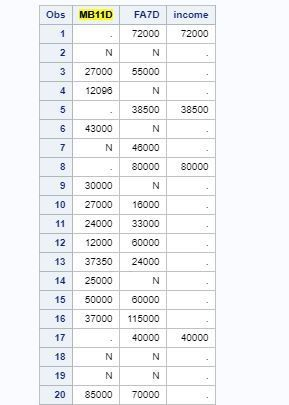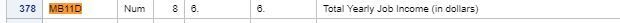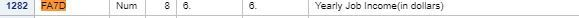## Sum command for numeric variables

Hello, I am trying to sum two numeric variables on SAS university.

I am using the code:

``income= sum(FA7D, MB11D);run;``

However, the results are not summing the numeric variables. I used the

proc print; command for my variables and this is what I am seeing...Can anyone please tell me what I am doing wrong?

Thanks,

1 ACCEPTED SOLUTION

Accepted Solutions

## Re: Sum command for numeric variables

Thank you for taking the time to respond to my message. I made a mistake in another part of my code, and that was why the sum command wasn't working as intended. I didn't want to post the entire code because I thought it was too long. Next time I will make sure to post everything.

Thanks again

8 REPLIES 8

## Re: Sum command for numeric variables

If you have N in your data your variables may not be numeric.

If you're trying to get the sum of a column the SUM() function isn't correct, that calculates the SUM for a specific row.

If you're trying to get the sum of a column use a Summary PROC such as PROC MEANS, SUMMARY or UNIVARIATE for starters.

Video tutorials are here:

video.sas.com

And specifically calculating summary statistics is here:

http://video.sas.com/detail/videos/sas-analytics-u/video/3306906230001/summary-statistics-using-sas-...

@stickm wrote:

Hello, I am trying to sum two numeric variables on SAS university.

I am using the code:

``income= sum(FA7D, MB11D);run;``

However, the results are not summing the numeric variables. I used the

proc print; command for my variables and this is what I am seeing...Can anyone please tell me what I am doing wrong?

Thanks,

## Re: Sum command for numeric variables

Thank you for the quick reply.

I believe both variables are numeric. When I used the

``proc contents;``

function it showed me:I am trying to create a new variable that combines the total value in each row for FA7D and MB11D.

Everything resource I have found has told me to use the newvariable=sum(var1, var2) command. However, when I perform it, it doesn't sum the values. It shows the new variable value as missing instead of being summed.

Thanks again,art297
Opal | Level 21

## Re: Sum command for numeric variables

I doubt if anyone can help unless you show the entire datastep you ran and post your dataset (not a picture of it)

Art, CEO, AnalystFinder.com

## Re: Sum command for numeric variables

Is you use of the SUM function embedded in a condition step of your logic, i.e. in an "if" statement    or a do group triggered by an if statement?

Show us the log for a more precise answer.

--------------------------
The hash OUTPUT method will overwrite a SAS data set, but not append. That can be costly. Consider voting for Add a HASH object method which would append a hash object to an existing SAS data set

Would enabling PROC SORT to simultaneously output multiple datasets be useful? Then vote for
Allow PROC SORT to output multiple datasets

--------------------------

## Re: Sum command for numeric variables

Post the full log of the run. I belirve the hint is there.

There is no syntax error in your code income = sum(FA7D, MB11D);

## Re: Sum command for numeric variables

Show your entire code. I do NOT believe that is only the result for the code snippet you have shown as SAS will convert character values to numeric and sum the result.

Example:

```data junk;
input x \$ y \$;
z = sum(x,y);
datalines;
1 2
N 3
4 5
6 N
;
proc print;
run;```

There will be notes in the log like:

```NOTE: Character values have been converted to numeric values at the places given by:
(Line):(Column).
```

saying the character variables were treated as numeric.

If the variables are truly numeric the only way to have N displayed would be to have the special missing .N (yes there is a period preceding the N) and the SUM will still work. HOWEVER if you used income = FA7D + MB11D; That would generate the output you show for Proc Print.

Perhaps the data step you used SUM in had another error and did not replace a data set created with the + sum for some reason. Which is why we say : Show the log of the data step with any messages. Paste into a code box opened with the forum {I} icon to preserve formatting of any error or warning messages.

## Re: Sum command for numeric variables

Thank you for taking the time to respond to my message. I made a mistake in another part of my code, and that was why the sum command wasn't working as intended. I didn't want to post the entire code because I thought it was too long. Next time I will make sure to post everything.

Thanks again

## Re: Sum command for numeric variables

Please mark this as resolved, you can use your last post as the correct solution.

Discussion stats
• 8 replies
• 4769 views
• 0 likes
• 6 in conversation